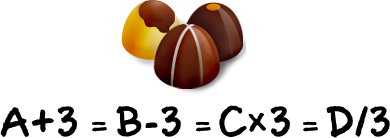## 四份巧克力數學謎題#### 解析

• A+3=B-3=C×3=D/3
• A+B+C+D=48

→ (E-3)+(E+3)+(E/3)+(E×3)=48
→ E=9

30,382